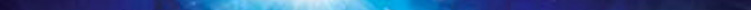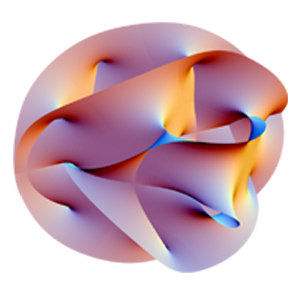Home Research Education and Outreach Job Openings News People Visiting Scholars Local EventsPhysicists and cosmologists spend a great deal of time wondering why the universe is so large. The distance scales that appear in our theories of physics, like the sizes of the atom or nucleus, are simply minute compared with the scale of the universe. One theory that provides an origin for the huge scale of the universe is inflation: the scale of the universe started small but then went through an era of exponential expansion. Another difficult question is why there are three dimensions to space. We have a hard time visualizing more than three dimensions to space, but this is just a limitation of our imagination, as many of our theories can be formulated in any number of dimensions. However, some theories are only mathematically consistent if space has a certain number of dimensions: for example, superstring theory needs nine spatial dimensions. It therefore seems very plausible that there are extra dimensions of space, and that we don't observe them in everyday life because they are small — they did not take part in inflation.String Theory predicts the existence of more than the 3 space dimensions and 1 time dimension we are all familiar with. According to string theory, there are additional dimensions that we are unfamiliar with because they are curled up into tiny complicated shapes that can only be seen on tiny scales. If we could shrink to this tiny, Planck-sized scale we could see that at every 3D point in space, we can also explore 6 additional dimensions. This animation shows an array of Calabi-Yau spaces which are projections of these higher dimensions into the more familiar dimensions we are aware of. Watch an explanation of strings on the NOVA presentation of Brian Greene's 'The Elegant Universe' - choose 'Multiple Dimensions'Theories predict a world of new dimensions   Supersymmetry predicts quantum dimensions connecting forces and matter Unification of gravity with the other forces requires dimensions beyond the three that we know Could there be extra time dimensions? Are we part of a multidimensional megaverse?   You may be skeptical that we would not have noticed an extra dimension even if it were small. Surely if there were a fourth dimension to move along we could "lose" things which drop down into it — energy and atoms could slip away from us. In fact the known laws of quantum mechanics prevent this. We cannot resolve anything in a small extra dimension unless we have a very high energy probe — in particle physics we always expect to be surprised by new and exciting phenomena as we probe nature with higher energies and therefore search at smaller distances. Indeed many experiments are underway to search for extra dimensions of space: if a high energy collision causes a quark to head off in an extra dimension it will appear to us as an excited state of the quark — it will look as if the quark has magically gained some mass. Other experiments look for a change in the Newtonian law of gravity at short distance. If the gravitational field has more dimensions to spread out in, then the gravitational force will vary more rapidly with distance than usual. The theoretical questions presented by the possibility of extra dimensions are both numerous and exciting. Discovery of extra dimensions would herald the first change in our view of spacetime since Einstein's theory of relativity. Since gravity is ultimately the geometry of spacetime, the theory of gravity is bound to be changed in spectacular ways. Perhaps it is the limitation to three spatial dimensions that prevents a reconciliation of gravity with quantum mechanics. Perhaps the resolution of the famous cosmological constant problem lies in extra dimensions. What is the fundamental law of physics which determines the size of these extra dimensions? Could it be that this is the scale of weak interactions? If so, perhaps extra dimensions hold the key to understanding the origin of how the weak gauge symmetry is broken, and how all the masses of the elementary particles are generated. Another possibility is that the size of the extra dimensions corresponds to the extremely high energy scale at which the strong, electromagnetic, and weak forces are unified into a single force. Such unified theories predict extremely rare decays of both the proton and the muon. Finally, perhaps the cosmological behavior of the universe at large distances is actually governed by the dynamics of the small extra dimensions. Inflation, and the observed dark matter and dark energies of the universe might all be manifestations of small extra dimensions. A remarkable connection between the very small and the very large! Contributed by Lawrence J. Hall ___________________________________________________________________________________Eleven dimensions, parallel universes, and a world made out of strings. It's not science fiction, it's String Theory. Watch the 3 episodes of The Elegant Universe produced by PBS.   ___________________________________________________________________________________   Strung Out on the Universe: Scientific American interviews Raphael Bousso about dark energy and string theory. Excerpt: "String theory offers a framework in which all forces are naturally, completely unified, including gravity. We really don't have anything else that achieves the same kind of unification. If you ask what string theory has done, for example, it describes in principle how the particles that make up gravity interact with each other. And it has explained in certain very special cases the entropy of black holes, which is a big problem in quantum gravity."   ___________________________________________________________________________________Calabi-Yau manifold Calabi-Yau manifolds are a special class of manifolds used in some branches of mathematics (such as algebraic geometry) as well as in theoretical physics. For instance, in superstring theory the extra dimensions of spacetime are sometimes conjectured to take the form of a 6-dimensional Calabi-Yau manifold. The precise definition of a Calabi-Yau manifold, given below, builds on a considerable mathematical background. The designation "Calabi-Yau space" for a member of this class was coined by physicists in the 1980s, but mathematicians have been studying such manifolds since at least the 1950s. Physical insights about Calabi-Yau manifolds, especially mirror symmetry, led to tremendous progress in pure mathematics. From wikipedia.